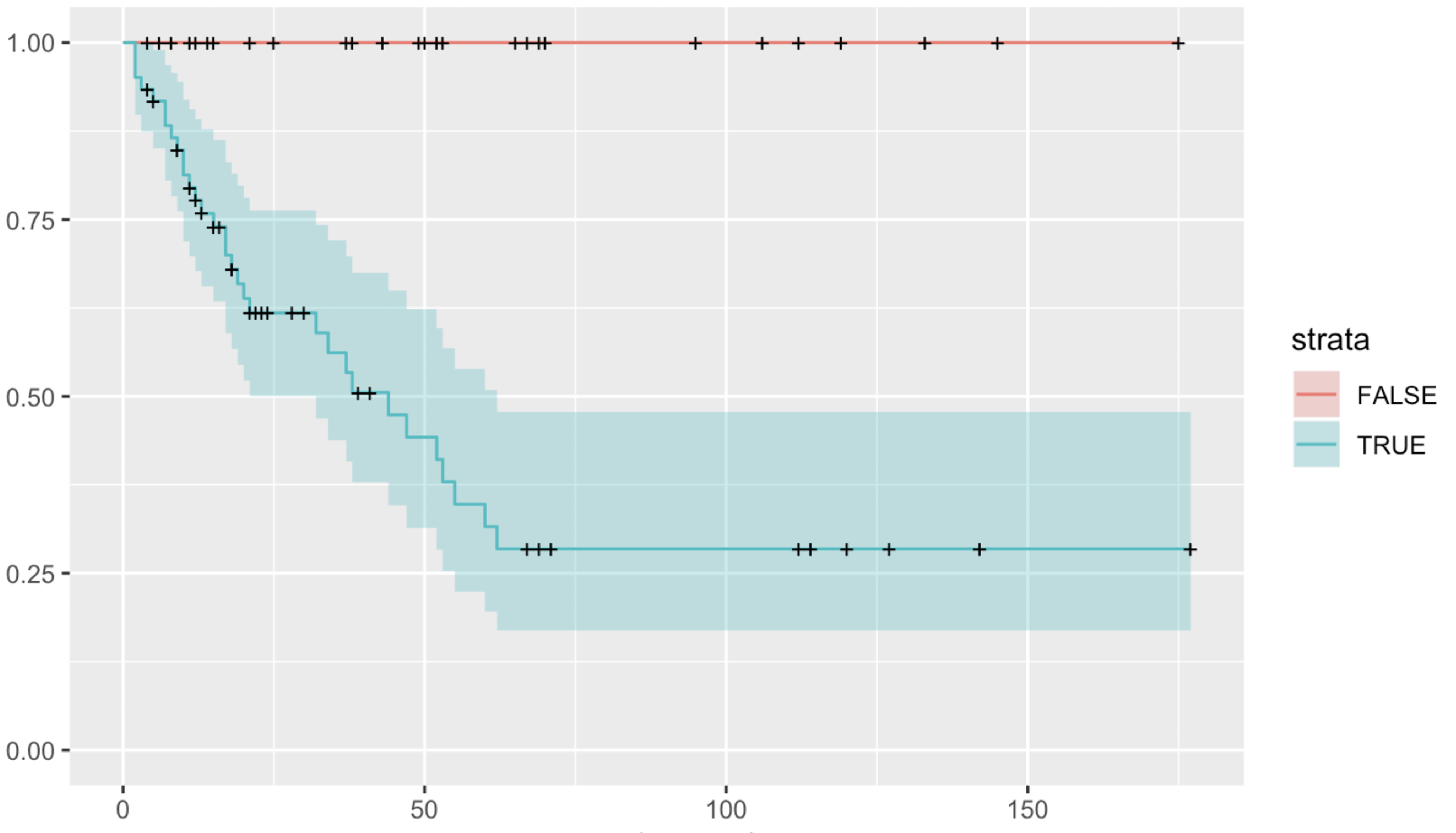Cox proportional hazards - how to interpret summary output
1
2
Entering edit mode
2.5 years ago

Hi all,

I've been using a cox proportional hazard model to do survival analysis in R. I am looking for some advice interpreting the p-values produced by this model. I came across the interesting case where I stratified my data into two groups and the survival curve looked like this:This was generated with code of the form shown below, where var is a binary variable:

km_fit <- survfit(Surv(time, status) ~ var, data=data)
cox <- coxph(Surv(time, status) ~ var, data=data)


When I run summary(cox) I get the following output:

Call:
cox <- coxph(Surv(time, status) ~ var, data=data)

n= 93, number of events= 32

coef exp(coef)  se(coef)     z Pr(>|z|)
varTRUE 1.991e+01 4.449e+08 4.441e+03 0.004    0.996

exp(coef) exp(-coef) lower .95 upper .95
varTRUE 444911382  2.248e-09         0       Inf

Concordance= 0.696  (se = 0.047 )
Rsquare= 0.305   (max possible= 0.935 )
Likelihood ratio test= 33.79  on 1 df,   p=6e-09
Wald test            = 0  on 1 df,   p=1
Score (logrank) test = 22.29  on 1 df,   p=2e-06


I was quite surprised that using a Wald test, there was no difference between the groups (p=1) - presumably due to the absence of events in one group. I wonder if anyone could advise me when it is appropriate to use each of these different statistical tests to generate a p-value? What are the underlying assumptions? Is there a good reference on this that you could direct me towards?

Many thanks

R • 3.2k views
ADD COMMENT
1
Entering edit mode
2.5 years ago

The wald test produced a p-value of 1 because the fit was really really poor. You can see that in the coef field, where it's 19.91+/-4441. That's then leading to the really low Z value (19.91/4441 ~= 0.004) and thus the poor p-value.

I don't know why the fit was so poor, but at least that's the reason for p=1.

ADD COMMENT
1
Entering edit mode

Indeed, when you are testing a dichotomous variable and one has no events, Wald test will invariably be 1 due to convergence, and should not be used. Take the log rank or LRT p-values.

ADD REPLY
0
Entering edit mode

Makes sense, see also here.

ADD REPLY
0
Entering edit mode

Confidence intervals on blue variable neither look great, though!

ADD REPLY

Login before adding your answer.

Traffic: 2423 users visited in the last hour
Help About
FAQ
Access RSS
API
Stats

Use of this site constitutes acceptance of our User Agreement and Privacy Policy.